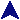Restack With Save And RestoreDr. Bennet# Tswitch

Main Page
F06 Assignment
CCSC '07 Paper

Save And Restore
Restack
Multiple Stacks

## Clients for the F06 Assignment

Simple
Graph Search
This demo shows how stack changes interact with register save and restore. Since the stack pointer is saved and restored, a `regrest` call can change which stack the CPU is using, which can change the values of variables stored on the stack. The output is:
A: arg = 15, r = 0
B: arg = 15
C: arg = 77
A: arg = 15, r = 1
E: arg = 15
D: arg = 77
E: arg = 77

```#include <stdio.h>
#include <stdlib.h>
#include <tswitch.h>

unsigned newstack1;
regbuf_t buf1, buf2;

{

int r = regsave(buf1);
printf("A: arg = %d, r = %d\n", arg, r);

if(r == 0) {
printf("B: arg = %d\n", arg);
restack(newstack1, sizeof newstack1, 2, 8);
// ** Now we're on the new stack.
arg = 77;
if(!regsave(buf2)) {
printf("C: arg = %d\n", arg);
regrest(buf1, 1); // ** Returns to old stack.
} else
// Here we're on the new stack, since regsave
// restored the stack pointer.
printf("D: arg = %d\n", arg);

}

// We're on the old stack the first time we pass this, and on the
// new stack the second time.
printf("E: arg = %d\n", arg);
return arg;
}

main()
{
int ret = egad(15);

printf("egad => %d\n", ret);

if(ret < 20) regrest(buf2, 2);

exit(0);
}
```